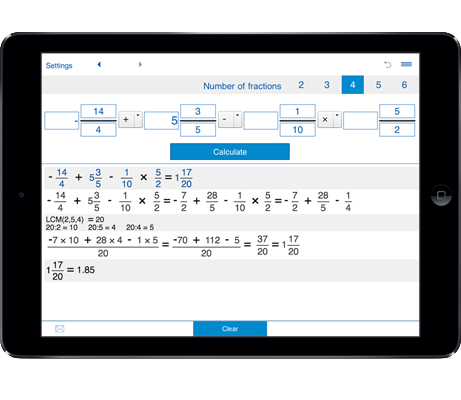Fraction calculator with steps for operations with two, three, four, five, and six fractions.
With fractions calculator you can add fractions with like and unlike denominators, add mixed fractions and whole numbers.

## Adding fractions calculator - add fractions, mixed fractions, and whole numbers

You can add up to six fractions.

### How to use the adding fractions calculator

1. Select the number of fractions you need to add - 2 fractions, 3 fractions, 4 fractions, 5 fractions, or 6 fractions.

2. Enter the fractions, mixed fractions or whole numbers you want to add.

For adding negative fractions put a minus sign.

3. Click the "Calculate" button.